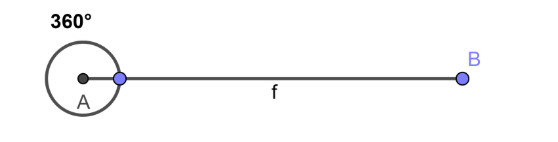QUESTION

# There are four angles formed at a point say, w, x, y, z. If w = x = y and z = 2x then find the value of w, x, y, z.A.$w=x=y=72{}^\circ \,\,\,\,z=144{}^\circ$B.$w=x=y=144{}^\circ \,\,\,\,z=72{}^\circ$C.$w=x=y=60{}^\circ \,\,\,\,z=120{}^\circ$D.$w=x=y=90{}^\circ \,\,\,\,z=180{}^\circ$

Hint: Use the concept “the sum of all the angles at a point is always equal to $360{}^\circ$” and convert the equation in terms of ‘x’ by using the given data and solve to get the value of ‘x’. Then use the value of ‘x’ to get the final answer.

To solve the above question we will write the given data first, therefore,
w = x = y and z = 2x ………………………………………………….. (1)
Now to proceed further in the solution we should know the concept given below,
Concept: The measure of angle at any point is $360{}^\circ$ and therefore the sum of all the angles at a point is always equal to $360{}^\circ$.
This concept can easily be understood from the diagram given below,If we refer to the above concept then we can say that the sum of w, x, y, and z is also equal to $360{}^\circ$ and therefore we can write,
$\Rightarrow w+x+y+z=360{}^\circ$
If we refer equation (1) then we know that w = x = y and therefore we can replace w and y by x, therefore we will get,
$\Rightarrow x+x+x+z=360{}^\circ$
Also if we put the value of z from equation (1) in the above equation we will get,
$\Rightarrow x+x+x+2x=360{}^\circ$
If we perform the addition operation in the above equation we will get,
$\Rightarrow 5x=360{}^\circ$
If we shift 5 on the right hand side of the equation we will get,
$\Rightarrow x=\dfrac{360{}^\circ }{5}$
If we divide $360{}^\circ$ by 5 in the above equation we will get,
$\Rightarrow x=72{}^\circ$
If we put this value in the equation (1) we will get,
w = $72{}^\circ$ = y And z = $2\times 72{}^\circ$
Therefore we can write,
w = x = y = $72{}^\circ$ And z = $144{}^\circ$
Therefore the value of w, x, y and z is given by $w=x=y=72{}^\circ \,\,\,\,z=144{}^\circ$.
Therefore the correct answer is option (a).

Note: In the equation $w+x+y+z=360{}^\circ$ we substitute one value for w, x, and y but we have to put this value as ‘x’ as the value of z is also in terms of ‘x’ and therefore it will be easy to form equation in one variable and solve for it.